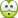# Hydrogen Atom Photon Emission Wavelength Formula

I am trying to calculate the Lyman-alpha wavelengths of photons emitted from different hydrogen-like atoms such as deuterium and positive helium ion 4He+, using the relation 1/λ = R*|1/ni^2 - 1/nf^2|, where R is the Rydberg constant and ni and nf are integer numbers corresponding to the initial and final energy levels, which, for Lyman-alpha wavelength, are 2 and 1, respectively. The expression for Rydberg constant is R = me/(4πħ3c)*(e2/4πε0)2.
My question is: what changes in these two expressions when we are dealing with different hydrogen-like atoms?replace electron mass me with new reduced mass μ? atomic number Z (but it is not included in any of the two expressions)?
Any help will be appreciated!ShayanJ
Gold Member
See here!
Thank you Shyan! I know now that you have to multiply by the atomic number Z squared but the site does not explain how the Rydberg constant changes for different elements, or does it even change?ShayanJ
Gold Member
Thank you Shyan! I know now that you have to multiply by the atomic number Z squared but the site does not explain how the Rydberg constant changes for different elements, or does it even change?It uses the same R in both equations!

It uses the same R in both equations!
Thank you!

It uses the same R in both equations!
Will there be no photon emitted if we are dealing with a positronium or muonium because there is no proton so the Z number is 0?

ShayanJ
Gold Member
Will there be no photon emitted if we are dealing with a positronium or muonium because there is no proton so the Z number is 0?
The Rydberg formula is an experimental result devised by observing the spectrum of different atoms in a time when muons and positrons(and actually even electrons) where not known! So, as it is, you can't expect it to describe the spectrum of particles you mentioned as well. Maybe using quantum mechanics it can be shown that it actually does work for the particles you mentioned but that's another matter.

jtbell
Mentor
Yes, the Rydberg constant was originally an empirical value, determined by fitting to the measured values of the wavelengths of the hydrogen spectrum. The major triumph of Bohr's atomic model was that it predicted the value of R from more fundamental constants, as given by the formula in the first post. It can also be derived from the solution of Schrödinger's equation for the hydrogen atom.

For positronium or muonium you have to replace me with the reduced mass of the system (electron+positron or muon+proton or whatever), like you do for any other hydrogen-like system like He+, Li++, etc. (or even plain hydrogen itself).

For multiple-charge nuclei you consider the e2 to be (ee)(enuc) and replace enuc = Zee, so e2 becomes Ze2.

The Rydberg formula is an experimental result devised by observing the spectrum of different atoms in a time when muons and positrons(and actually even electrons) where not known! So, as it is, you can't expect it to describe the spectrum of particles you mentioned as well. Maybe using quantum mechanics it can be shown that it actually does work for the particles you mentioned but that's another matter.
Thank you Shyan!

Yes, the Rydberg constant was originally an empirical value, determined by fitting to the measured values of the wavelengths of the hydrogen spectrum. The major triumph of Bohr's atomic model was that it predicted the value of R from more fundamental constants, as given by the formula in the first post. It can also be derived from the solution of Schrödinger's equation for the hydrogen atom.

For positronium or muonium you have to replace me with the reduced mass of the system (electron+positron or muon+proton or whatever), like you do for any other hydrogen-like system like He+, Li++, etc. (or even plain hydrogen itself).

For multiple-charge nuclei you consider the e2 to be (ee)(enuc) and replace enuc = Zee, so e2 becomes Ze2.
That explains a lot! Thank you jtbell!

Khashishi
It uses the same R in both equations!
You seem to imply that R doesn't depend on element or isotope, which is wrong. Maybe you meant something different, but I can see this statement confusing the OP, so I have to respond.

If you look here, it shows you that you have to make an adjustment for the reduced mass of the system.
https://en.wikipedia.org/wiki/Rydberg_constant
$$R_M = \frac{1}{1+m_e/M} R_\infty$$
M is the mass of the nucleus. Or in the case of positronium, M=m_e, so the Rydberg constant is approximately 1/2 of hydrogen. (This works out to the same as replacing ##m_e## by ##\mu##)

You seem to imply that R doesn't depend on element or isotope, which is wrong. Maybe you meant something different, but I can see this statement confusing the OP, so I have to respond.

If you look here, it shows you that you have to make an adjustment for the reduced mass of the system.
https://en.wikipedia.org/wiki/Rydberg_constant
$$R_M = \frac{1}{1+m_e/M} R_\infty$$
M is the mass of the nucleus. Or in the case of positronium, M=m_e, so the Rydberg constant is approximately 1/2 of hydrogen. (This works out to the same as replacing ##m_e## by ##\mu##)

Thank you for your help Khashishi!